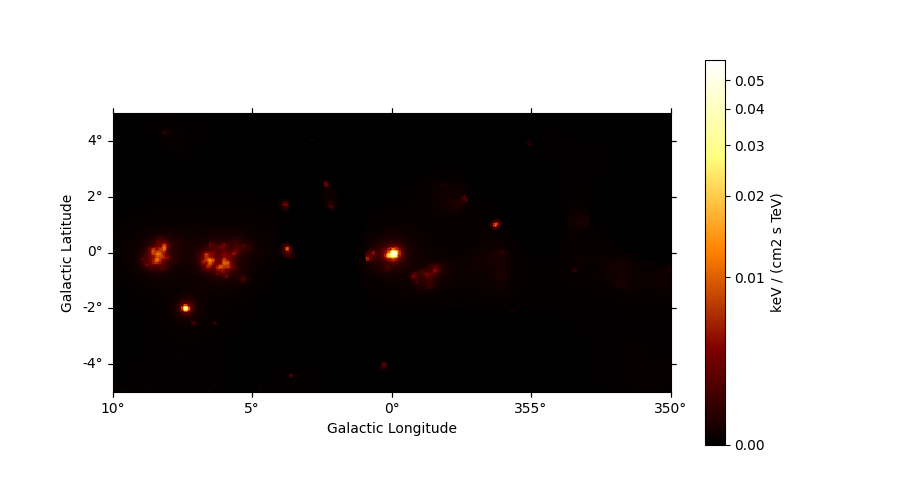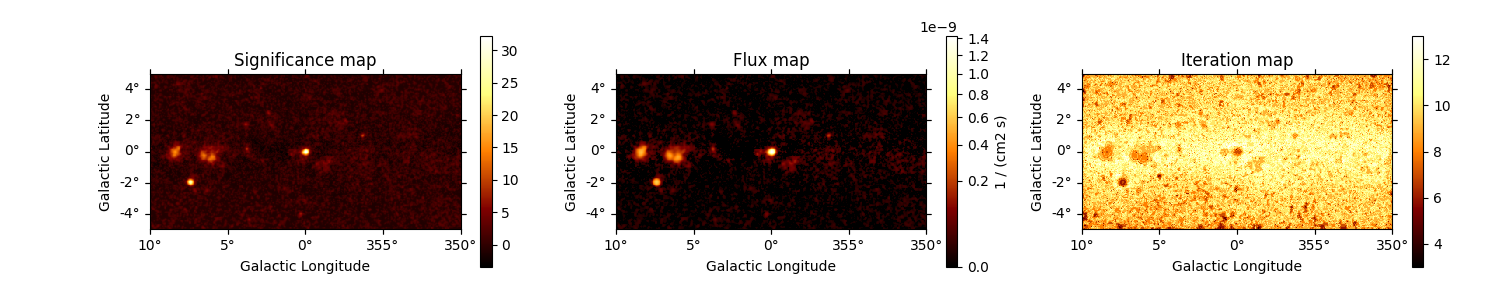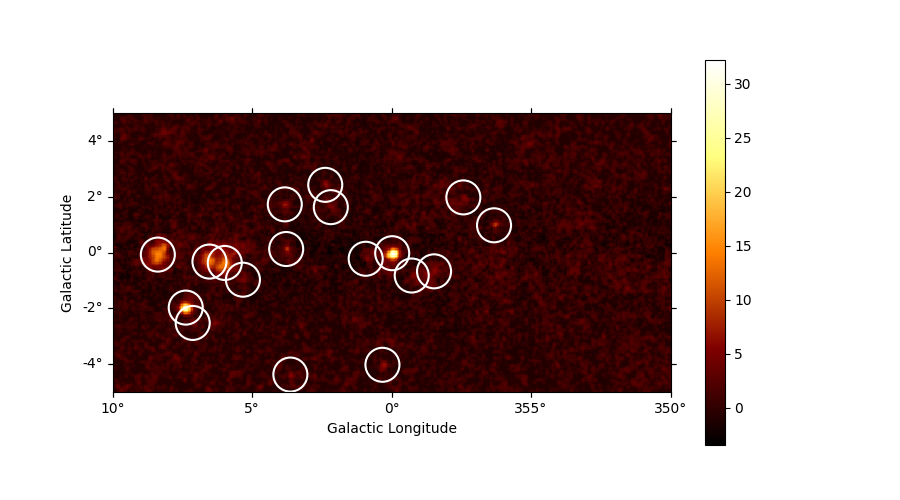# Source detection and significance maps#

Build a list of significant excesses in a Fermi-LAT map.

## Context#

The first task in a source catalogue production is to identify significant excesses in the data that can be associated to unknown sources and provide a preliminary parametrization in term of position, extent, and flux. In this notebook we will use Fermi-LAT data to illustrate how to detect candidate sources in counts images with known background.

Objective: build a list of significant excesses in a Fermi-LAT map

## Proposed approach#

This notebook show how to do source detection with Gammapy using the methods available in `estimators`. We will use images from a Fermi-LAT 3FHL high-energy Galactic center dataset to do this:

• perform adaptive smoothing on counts image

• produce 2-dimensional test-statistics (TS)

• run a peak finder to detect point-source candidates

• compute Li & Ma significance images

• estimate source candidates radius and excess counts

Note that what we do here is a quick-look analysis, the production of real source catalogs use more elaborate procedures.

We will work with the following functions and classes:

## Setup#

As always, let’s get started with some setup …

```import numpy as np
import astropy.units as u

# %matplotlib inline
import matplotlib.pyplot as plt
from IPython.display import display
from gammapy.datasets import MapDataset
from gammapy.estimators import ASmoothMapEstimator, TSMapEstimator
from gammapy.estimators.utils import find_peaks
from gammapy.irf import EDispKernelMap, PSFMap
from gammapy.maps import Map
from gammapy.modeling.models import PointSpatialModel, PowerLawSpectralModel, SkyModel
```

## Check setup#

```from gammapy.utils.check import check_tutorials_setup

check_tutorials_setup()
```
```System:

python_executable      : /home/runner/work/gammapy-docs/gammapy-docs/gammapy/.tox/build_docs/bin/python
python_version         : 3.9.15
machine                : x86_64
system                 : Linux

Gammapy package:

version                : 1.0
path                   : /home/runner/work/gammapy-docs/gammapy-docs/gammapy/.tox/build_docs/lib/python3.9/site-packages/gammapy

Other packages:

numpy                  : 1.23.4
scipy                  : 1.9.3
astropy                : 5.1.1
regions                : 0.7
click                  : 8.1.3
yaml                   : 6.0
IPython                : 8.6.0
jupyterlab             : not installed
matplotlib             : 3.6.2
pandas                 : not installed
healpy                 : 1.16.1
iminuit                : 2.17.0
sherpa                 : 4.15.0
naima                  : 0.10.0
emcee                  : 3.1.3
corner                 : 2.2.1

Gammapy environment variables:

GAMMAPY_DATA           : /home/runner/work/gammapy-docs/gammapy-docs/gammapy-datasets/1.0
```

We first read the relevant maps:

```counts = Map.read("\$GAMMAPY_DATA/fermi-3fhl-gc/fermi-3fhl-gc-counts-cube.fits.gz")
"\$GAMMAPY_DATA/fermi-3fhl-gc/fermi-3fhl-gc-background-cube.fits.gz"
)

"\$GAMMAPY_DATA/fermi-3fhl-gc/fermi-3fhl-gc-psf-cube.fits.gz",
format="gtpsf",
)

edisp = EDispKernelMap.from_diagonal_response(
energy_axis=counts.geom.axes["energy"],
energy_axis_true=exposure.geom.axes["energy_true"],
)

dataset = MapDataset(
counts=counts,
background=background,
exposure=exposure,
psf=psfmap,
name="fermi-3fhl-gc",
edisp=edisp,
)
```
```/home/runner/work/gammapy-docs/gammapy-docs/gammapy/.tox/build_docs/lib/python3.9/site-packages/astropy/wcs/wcs.py:725: FITSFixedWarning: 'datfix' made the change 'Set DATEREF to '2001-01-01T00:01:04.184' from MJDREF.
Set MJD-OBS to 54682.655283 from DATE-OBS.
Set MJD-END to 57236.967546 from DATE-END'.
warnings.warn(
```

For visualisation purpose it can be nice to look at a smoothed counts image. This can be performed using the adaptive smoothing algorithm from Ebeling et al. (2006).

In the following example the `ASmoothMapEstimator.threshold` argument gives the minimum significance expected, values below are clipped.

```scales = u.Quantity(np.arange(0.05, 1, 0.05), unit="deg")
smooth = ASmoothMapEstimator(threshold=3, scales=scales, energy_edges=[10, 500] * u.GeV)
images = smooth.run(dataset)

plt.figure(figsize=(9, 5))
``````<WCSAxesSubplot: >
```

## TS map estimation#

The Test Statistic, TS = 2 ∆ log L (Mattox et al. 1996), compares the likelihood function L optimized with and without a given source. The TS map is computed by fitting by a single amplitude parameter on each pixel as described in Appendix A of Stewart (2009). The fit is simplified by finding roots of the derivative of the fit statistics (default settings use Brent’s method).

We first need to define the model that will be used to test for the existence of a source. Here, we use a point source.

```spatial_model = PointSpatialModel()

# We choose units consistent with the map units here...
spectral_model = PowerLawSpectralModel(amplitude="1e-22 cm-2 s-1 keV-1", index=2)
model = SkyModel(spatial_model=spatial_model, spectral_model=spectral_model)
```
```estimator = TSMapEstimator(
model,
kernel_width="1 deg",
energy_edges=[10, 500] * u.GeV,
)
maps = estimator.run(dataset)
```

### Plot resulting images#

```fig, (ax1, ax2, ax3) = plt.subplots(
ncols=3,
figsize=(15, 3),
subplot_kw={"projection": counts.geom.wcs},
gridspec_kw={"left": 0.1, "right": 0.98},
)

ax1.set_title("Significance map")
ax2.set_title("Flux map")
ax3.set_title("Iteration map")
``````Text(0.5, 1.0, 'Iteration map')
```

## Source candidates#

Let’s run a peak finder on the `sqrt_ts` image to get a list of point-sources candidates (positions and peak `sqrt_ts` values). The `find_peaks` function performs a local maximum search in a sliding window, the argument `min_distance` is the minimum pixel distance between peaks (smallest possible value and default is 1 pixel).

```sources = find_peaks(maps["sqrt_ts"], threshold=5, min_distance="0.25 deg")
nsou = len(sources)
display(sources)

# Plot sources on top of significance sky image
plt.figure(figsize=(9, 5))

ax.scatter(
sources["ra"],
sources["dec"],
transform=plt.gca().get_transform("icrs"),
color="none",
edgecolor="w",
marker="o",
s=600,
lw=1.5,
)
plt.show()

# sphinx_gallery_thumbnail_number = 3
``````value   x   y      ra       dec
deg       deg
------ --- --- --------- ---------
32.206 200  99 266.41449 -28.97054
27.836  52  60 272.43197 -23.54282
15.171  32  98 271.16056 -21.74479
14.143  69  93 270.40919 -23.47797
13.882  80  92 270.15899 -23.98049
9.7642 273 119 263.18257 -31.52587
8.7947 124 102 268.46711 -25.63326
7.3501 123 134 266.97596 -24.77174
6.8086 193  19 270.59696 -30.69138
6.2444 152 148 265.48068 -25.64323
5.8767 230  86 266.15140 -30.58926
5.6659 127  12 272.77351 -27.97934
5.6556 251 139 262.90685 -30.05853
5.4732 181  95 267.17020 -28.26173
5.4236 214  83 266.78188 -29.98429
5.1755  57  49 272.82739 -24.02653
5.0674 156 132 266.12148 -26.23306
5.0447  93  80 270.37773 -24.84233
```

Note that we used the instrument point-spread-function (PSF) as kernel, so the hypothesis we test is the presence of a point source. In order to test for extended sources we would have to use as kernel an extended template convolved by the PSF. Alternatively, we can compute the significance of an extended excess using the Li & Ma formalism, which is faster as no fitting is involve.

## What next?#

In this notebook, we have seen how to work with images and compute TS and significance images from counts data, if a background estimate is already available.

Here’s some suggestions what to do next:

Total running time of the script: ( 0 minutes 15.551 seconds)

Gallery generated by Sphinx-Gallery